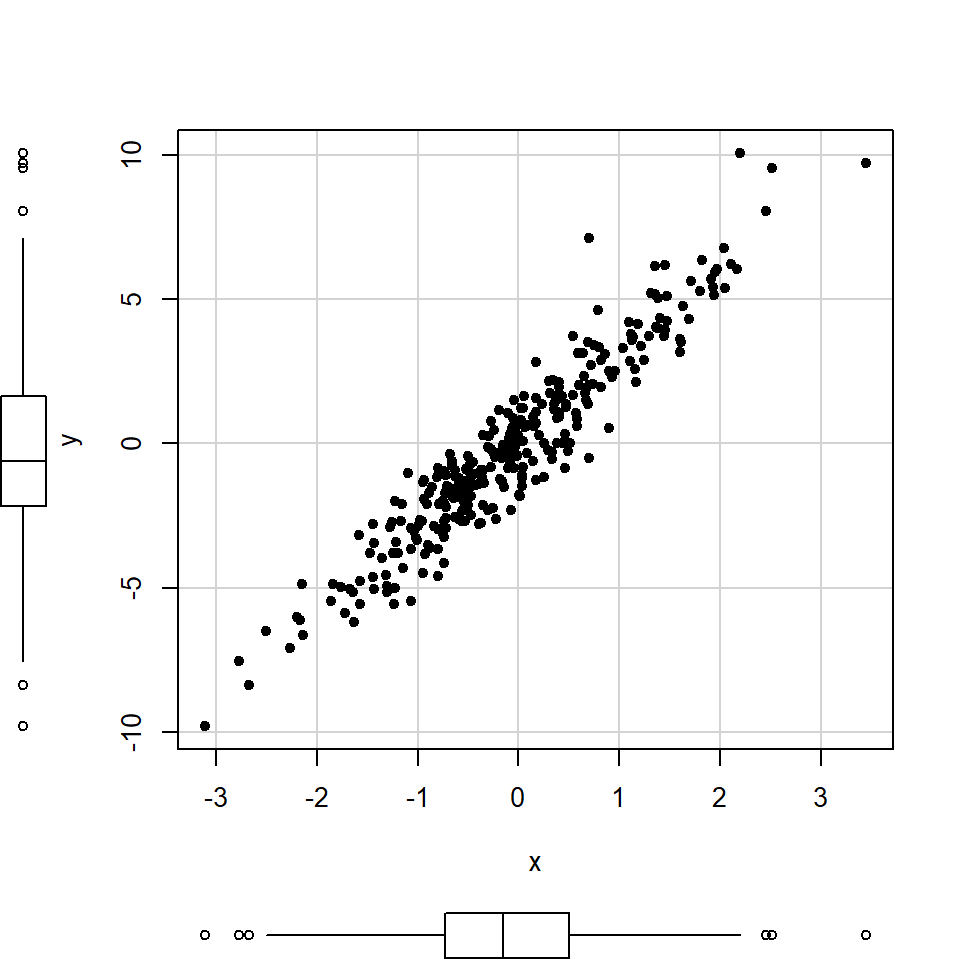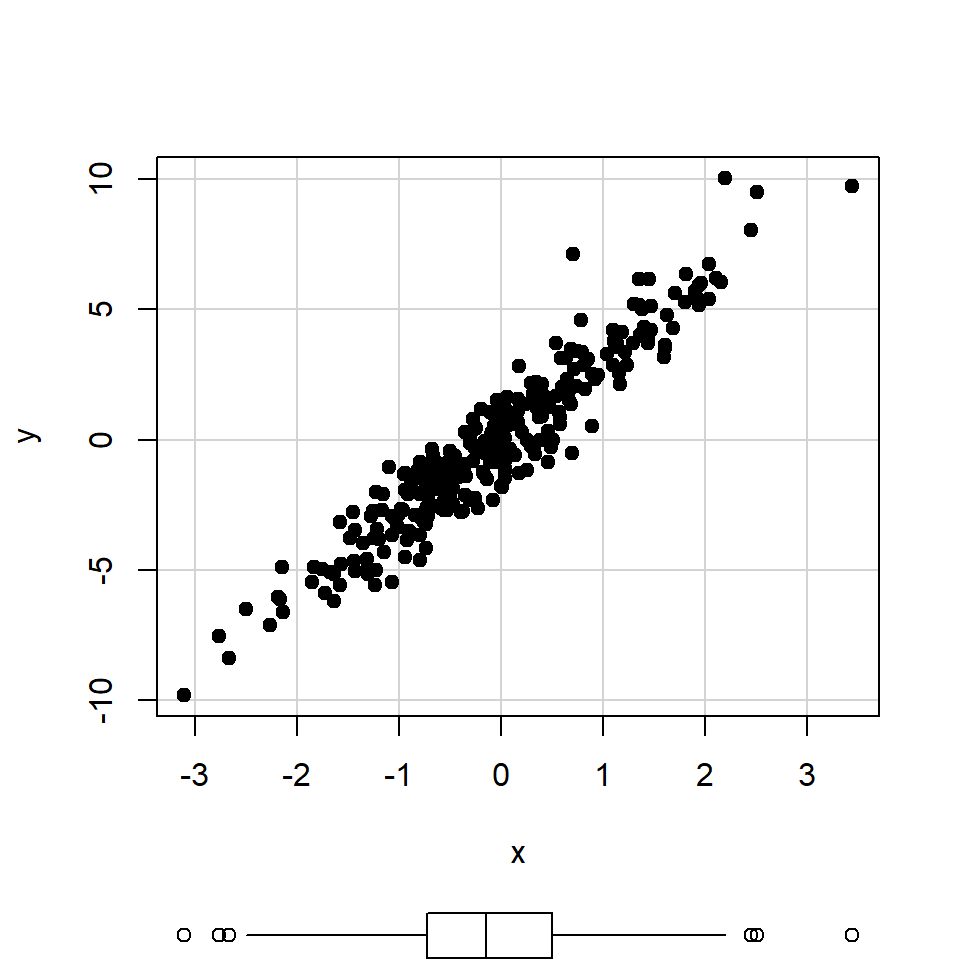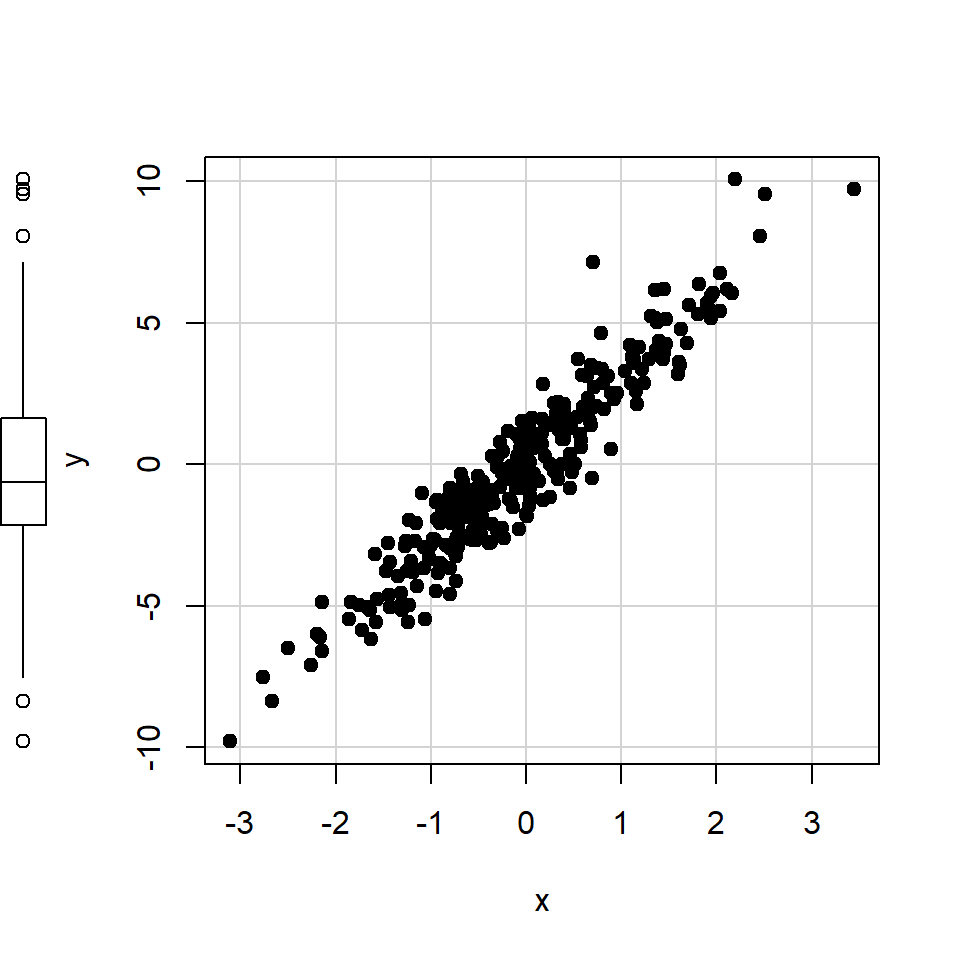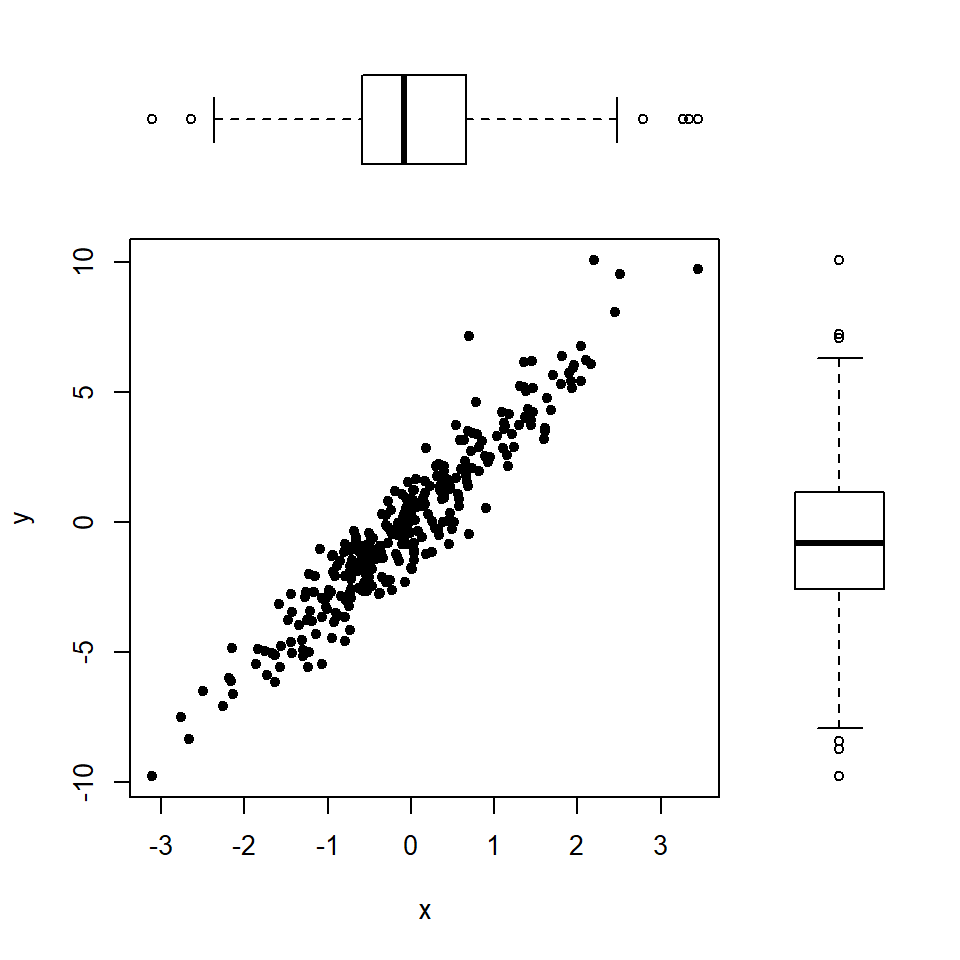# Scatter plot with marginal box plots in R

John Fox

## `scatterplot` function

The `car` package contains a function named `scatterplot` which creates scatter plots in base R with marginal box plots. Note that by default the function also adds smoothed and linear regression lines, which we omitted.

Scatter plot with marginal box plots

``````# install.packages("car")
library(car)

# Data
set.seed(14022021)
x <- rnorm(300)
y <- 3 * x + rnorm(300)

scatterplot(x, y,            # Data
pch = 19,        # Symbol of the points
col = 1,         # Color of the points
smooth = FALSE,  # Remove smooth estimate
regLine = FALSE) # Remove linear estimate``````The `boxplots` argument of the function defaults to `"xy"`. However, if you set `boxplots = "x"` only the box plot for the X-axis will be displayed.

``````# install.packages("car")
library(car)

# Data
set.seed(14022021)
x <- rnorm(300)
y <- 3 * x + rnorm(300)

scatterplot(x, y,            # Data
boxplots = "x",  # Box plot on the X-axis
pch = 19,        # Symbol of the points
col = 1,         # Color of the points
smooth = FALSE,  # Remove smooth estimate
regLine = FALSE) # Remove linear estimate``````Equivalently, if you set `boxplots = "y"` only the Y-axis box plot will be drawn.

``````# install.packages("car")
library(car)

# Data
set.seed(14022021)
x <- rnorm(300)
y <- 3 * x + rnorm(300)

scatterplot(x, y,            # Data
boxplots = "y",  # Box plot on the Y-axis
pch = 19,        # Symbol of the points
col = 1,         # Color of the points
smooth = FALSE,  # Remove smooth estimate
regLine = FALSE) # Remove linear estimate``````## Using the `layout` function

The function of the last section is very useful for adding marginal box plots quickly. However, it doesn’t allow further customization of the box plots or change the position of the box plots.

The `layout` function can be used to add marginal charts, allowing you to customize each plot and placing it where you want. If you are not familiar with this function check the tutorial about combining plots.``````# Data
set.seed(14022021)
x <- rnorm(300)
y <- 3 * x + rnorm(300)

# Layout
layout(matrix(c(2, 0, 1, 3),
nrow = 2, ncol = 2,
byrow = TRUE),
widths = c(3, 1),
heights  = c(1, 3), respect = TRUE)

# Top and right margin of the main plot
par(mar = c(5.1, 4.1, 0, 0))
plot(x, y, pch = 19, col = 1, cex = 0.8)

# Left margin of the top box plot
par(mar = c(0, 4.1, 0, 0), bty = "n")
boxplot(y, axes = FALSE, horizontal = TRUE,
col = "white")

# Bottom margin of the right box plot
par(mar = c(5.1, 0, 0, 0), bty = "n")
boxplot(x, axes = FALSE, col = "white")``````